# Substitution

solve equations by substitution:

x+y= 11
y=5x-25

x =  6
y =  5

### Step-by-step explanation:

x+y= 11
y=5x-25

x+y= 11
y=5•x-25

x+y = 11
5x-y = 25

x = 6
y = 5

Our linear equations calculator calculates it.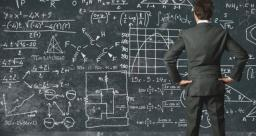Did you find an error or inaccuracy? Feel free to write us. Thank you!Tips to related online calculators
Do you have a linear equation or system of equations and looking for its solution? Or do you have a quadratic equation?

## Related math problems and questions:

• Two equationsSolve equations (use adding and subtracting of linear equations): -4x+11y=5 6x-11y=-5
• EquationsSolve following system of equations: 6(x+7)+4(y-5)=12 2(x+y)-3(-2x+4y)=-44
• Simple equation 324 = n • 27, solve for n
• Equations - simpleSolve system of linear equations: x-2y=6 3x+2y=4
• Rings groups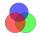27 pupils attend some group; dance group attends 14 pupils, 21 pupils sporty group and dramatic group 16 pupils. Dance and sporting attend 9 pupils, dance and drama 6 pupil, sporty and dramatic 11 pupils. How many pupils attend all three groups?
• TestingStudents of high school have 10 points for each good solved task. The wrong answer is deducted by 5 points. After solving 20 tasks, student Michael had 80 points. How many tasks did he solve correctly and how many wrong?
• Linear systemSolve a set of two equations of two unknowns: 1.5x+1.2y=0.6 0.8x-0.2y=2
• ATC campThe owner of the campsite offers 79 places in 22 cabins. How many of them are triple and quadruple?
• Trapezoidtrapezoid ABCD a = 35 m, b=28 m c = 11 m and d = 14 m. How to calculate its area?
• Coffee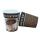Coffee from the machine in the cup cost 28 cents. Coffee is 20 cents more expensive than the cup. How much is the cup?
• Team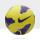The football team has 11 players. Their average age is 20 years. During the match due to injury, one player withdraws and the average age of the team is 18. How old was injured player?
• Freight train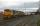The train carries 525 tons of limestone in 29 wagons. Wagons are 15 tonne and 20 tons. How many is 15 ton and how many is 20 ton wagons?
• Simple equationsSolve system of equations: 5x+3y=5 5x+7y=25
• Algebrogram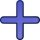Solve algebrogram: mama+anna=vari How many solutions are?
• AlgebrogramSolve algebrogram for sum of three numbers: BEK KEMR SOMR ________ HERCI
• Three unknownsSolve the system of linear equations with three unknowns: A + B + C = 14 B - A - C = 4 2A - B + C = 0
• Equation 29Solve next equation: 2 ( 2x + 3 ) = 8 ( 1 - x) -5 ( x -2 )